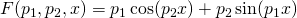Simple nonlinear least squares curve fitting in Mathematica

December 6th, 2013 | Categories: math software, mathematica, programming | Tags:

A question I get asked a lot is ‘How can I do nonlinear least squares curve fitting in X?’ where X might be MATLAB, Mathematica or a whole host of alternatives.  Since this is such a common query, I thought I’d write up how to do it for a very simple problem in several systems that I’m interested in

This is the Mathematica version. For other versions,see the list below

The problem

You have the following 10 data points

xdata = -2,-1.64,-1.33,-0.7,0,0.45,1.2,1.64,2.32,2.9
ydata = 0.699369,0.700462,0.695354,1.03905,1.97389,2.41143,1.91091,0.919576,-0.730975,-1.42001

and you’d like to fit the functionusing nonlinear least squares.  You’re starting guesses for the parameters are p1=1 and P2=0.2

For now, we are primarily interested in the following results:

• The fit parameters
• Sum of squared residuals

Future updates of these posts will show how to get other results such as confidence intervals. Let me know what you are most interested in.

Mathematica Solution using FindFit
FindFit is the basic nonlinear curve fitting routine in Mathematica

xdata={-2,-1.64,-1.33,-0.7,0,0.45,1.2,1.64,2.32,2.9};
ydata={0.699369,0.700462,0.695354,1.03905,1.97389,2.41143,1.91091,0.919576,-0.730975,-1.42001};

(*Mathematica likes to have the data in the form {{x1,y1},{x2,y2},..}*)
data = Partition[Riffle[xdata, ydata], 2];

FindFit[data, p1*Cos[p2 x] + p2*Sin[p1 x], {{p1, 1}, {p2, 0.2}}, x]

Out:={p1->1.88185,p2->0.70023}

Mathematica Solution using NonlinearModelFit
You can get a lot more information about the fit using the NonLinearModelFit function

(*Set up data as before*)
xdata={-2,-1.64,-1.33,-0.7,0,0.45,1.2,1.64,2.32,2.9};
ydata={0.699369,0.700462,0.695354,1.03905,1.97389,2.41143,1.91091,0.919576,-0.730975,-1.42001};
data = Partition[Riffle[xdata, ydata], 2];

(*Create the NonLinearModel object*)
nlm = NonlinearModelFit[data, p1*Cos[p2 x] + p2*Sin[p1 x], {{p1, 1}, {p2, 0.2}}, x];

The NonLinearModel object contains many properties that may be useful to us. Here’s how to list them all

nlm["Properties"]

Out= {"AdjustedRSquared", "AIC", "AICc", "ANOVATable", \
"ANOVATableDegreesOfFreedom", "ANOVATableEntries", "ANOVATableMeanSquares", \
"ANOVATableSumsOfSquares", "BestFit", "BestFitParameters", "BIC", \
"CorrelationMatrix", "CovarianceMatrix", "CurvatureConfidenceRegion", "Data", \
"EstimatedVariance", "FitCurvatureTable", "FitCurvatureTableEntries", \
"FitResiduals", "Function", "HatDiagonal", "MaxIntrinsicCurvature", \
"MaxParameterEffectsCurvature", "MeanPredictionBands", \
"MeanPredictionConfidenceIntervals", "MeanPredictionConfidenceIntervalTable", \
"MeanPredictionConfidenceIntervalTableEntries", "MeanPredictionErrors", \
"ParameterBias", "ParameterConfidenceIntervals", \
"ParameterConfidenceIntervalTable", \
"ParameterConfidenceIntervalTableEntries", "ParameterConfidenceRegion", \
"ParameterErrors", "ParameterPValues", "ParameterTable", \
"ParameterTableEntries", "ParameterTStatistics", "PredictedResponse", \
"Properties", "Response", "RSquared", "SingleDeletionVariances", \
"SinglePredictionBands", "SinglePredictionConfidenceIntervals", \
"SinglePredictionConfidenceIntervalTable", \
"SinglePredictionConfidenceIntervalTableEntries", "SinglePredictionErrors", \
"StandardizedResiduals", "StudentizedResiduals"}

Let’s extract the fit parameters, 95% confidence levels and residuals

{params, confidenceInt, res} =
nlm[{"BestFitParameters", "ParameterConfidenceIntervals", "FitResiduals"}]

Out= {{p1 -> 1.88185,
p2 -> 0.70023}, {{1.8186, 1.9451}, {0.679124,
0.721336}}, {-0.0276906, -0.0322944, -0.0102488, 0.0566244,
0.0920392, 0.0976307, 0.114035, 0.109334, 0.0287154, -0.0700442}}

The parameters are given as replacement rules. Here, we convert them to pure numbers

{p1, p2} = {p1, p2} /. params

Out= {1.88185,0.70023}

Although only a few decimal places are shown, p1 and p2 are stored in full double precision. You can see this by converting to InputForm

InputForm[{p1, p2}]

Out//InputForm=
{1.8818508498053645, 0.7002298171759191}

Similarly, let’s look at the 95% confidence interval, extracted earlier, in full precision

confidenceInt // InputForm

Out//InputForm=
{{1.8185969887307214, 1.9451047108800077},
{0.6791239458086734, 0.7213356885431649}}

Calculate the sum of squared residuals

resnorm = Total[res^2]

Out= 0.0538127

Notes
I used Mathematica 9 on Windows 7 64bit to perform these calculations

1. Thanks for this! For zippering up the data I often like to also use Transpose[{xdata, ydata}] which I think of as being like pythons “zip”.

2. I usually do the Riffle part like this:

Transpose[{xdata, ydata}]

It’s very efficient as it doesn’t unpack packed arrays. One could use Thread[] in place of Transpose[], but Thread[] unpacks. Thread[] is still useful for things like Thread[{{1, 2, 3, 4, 5}, 0}]

3. This Mathematica.SE thread might be useful if you need to change the default method used by FindFit or NonlinearModelFit: http://mathematica.stackexchange.com/q/21823/12

4. Thanks for that extra info guys.